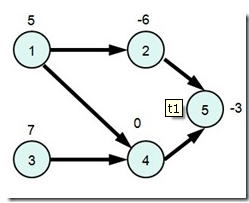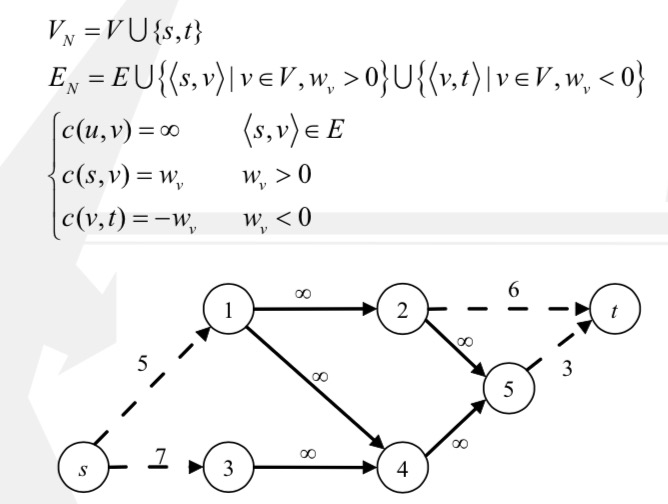# 最大权闭合图

## 据说这玩意算图论？# Maximum Weight Closure of a Graph

## 最大权闭合图{5},{4,5},{2,5},
{1,2,4,5},{1,2,3,4,5},
{2,4,5},{3,4,5}### 简单割

wft?为什么

$W(V_1)=\sum _{v\in V_1^+}W_v\ -\ \sum_{v\in V_1^-}(-W_v)$

$c[S,T]=\sum_{v\in V_2^+}W_v\ +\ \sum_{v\in V_1^-}(-W_v)$

$W(V_1)+c[S,T]=\sum _{v\in V_1^+}W_v\ -\ \sum_{v\in V_1^-}(-W_v)+\sum_{v\in V_2^+}W_v\ +\ \sum_{v\in V_1^-}(-W_v)$

$W(V_1)+c[S,T]=\sum _{v\in V_1^+}W_v+\sum_{v\in V_2^+}W_v$

$W(V_1)+c[S,T]=\sum _{v\in V^+}W_v$

$W(V_1)=\sum _{v\in V^+}W_v-c[S,T]$

By:Wahacer

2017.12.22

22:45